# Price discrimination requires market power

## Statement

One of the necessary conditions to sustain price discrimination as a profit-maximizing strategy is some degree of market power, i.e., the ability to set prices in the market because of the absence of competitors who can produce similar goods at similar costs of production.

The rough reason for this is that a strategy of price discrimination means that the most profitable customers are the most overcharged -- and hence, they can be most easily taken away by competitors.

## Example

Suppose a seller produces a good at a price of$x$ money units per piece, and there is no competition. Buyer$A$ is willing to pay a maximum of$y$ units for the good, and buyer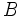$B$ is willing to pay$z$ units for the good. In other words, the reservation prices of buyers$A$ and$B$ are$y$ and$z$ units respectively.

We first consider the case where$\! z > y > x$ and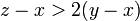$\! z - x > 2(y - x)$. For instance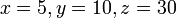$\! x = 5, y = 10, z = 30$.

Situation How the seller acts and buyers respond Producer surplus Consumer surplus Social surplus
Single price chosen by seller for all buyers (no price discrimination) Seller prices good at$z$,$B$ buys,$A$ doesn't.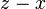$\! z - x$$\! 0$$\! z - x$
Separate price for each buyer Seller prices at$y$ for$A$ and$z$ for$B$, both buy.$\! y + z - 2x$$\! 0$$\! y + z - 2x$

Now, suppose a new seller enters the market. For simplicity, assume that this new seller has the same production costs ($x$ per unit). This seller can undercut the first seller on the price offered to$B$, by offering a price slightly less than$z$. Buyer$B$ shifts to the new seller, while$A$ (the less profitable customer) remains with the old seller. Thus, the new seller is now getting a profit close to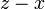$z - x$, more than the old seller's profit of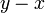$y - x$.

This forces the old seller to push down the price for$B$ to a level a little lower than the new seller. This competitive process continues till the prices drop -- they are likely to drop down at least to$y$, and in a perfectly competitive market, they may drop down all the way to$x$.

Note the important point that in order for the first seller to abandon the price discrimination strategy, it is not necessary for the new seller to price discriminate. In fact, the new seller concentrates on the highest reservation price buyers of the old seller by setting a uniform price that is (initially) less than the reservation price only for the buyers with the highest reservation price.

## Alternative explanations for price discrimination surviving without market power

In case of situations where there is evidence of a robust competitive market and price discrimination seems to persist despite that, there could be two explanations:

• There are genuine differences in the costs of serving the different buyers.
• The price discrimination is carried out to achieve goals other than immediate profit maximization. For instance, in some cases, lower prices for underdog customers may be used as a goodwill point for advertising and brand-building. In other cases, the lower prices may be a reflection of the beliefs and tastes of the business. The point here is that the sellers do pay a price for price discrimination as far as those specific transactions are concerned, and the compensating benefits (whether the private satisfaction of sellers or the long-term effects of brand-building) are harder to predict and discern.